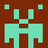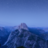首页   注册   登录
V2EX = way to explore
V2EX 是一个关于分享和探索的地方

Meteor
JSLint - a JavaScript code quality tool
jsFiddle
D3.js
WebStorm

JavaScript 权威指南第 5 版Closure: The Definitive GuideV2EX  ›  JavaScript

# 由 left-pad 扯到 JS 中的位运算

liuxin5959 · 2017-10-08 22:03:15 +08:00 · 1502 次点击

``````module.exports = leftpad;

function leftpad (str, len, ch) {
str = String(str);

var i = -1;

if (!ch && ch !== 0) ch = ' ';

len = len - str.length;

while (++i < len) {
str = ch + str;
}

return str;
}
``````

``````module.exports = leftpad;

function leftpad (str, len, ch) {
//convert the `str` to String
str = str +'';

len = len - str.length;
if (len <= 0) return str;

//convert the `ch` to String
if (!ch && ch !== 0) ch = ' ';
ch = ch + '';

while (true) {
if (len & 1) pad += ch;
len >>= 1;
if (len) ch += ch;
else break;
}
}
``````

### 原码、补码和反码

#### 反码

``````[+3]=原=反
[-3]=原=反
``````

#### 补码

``````[+3]=原=反=补
[-3]=原=反=补
``````

### 二进制与十进制的转换

#### 十进制小数转二进制

``````如:0.25 的二进制
0.25*2=0.5 取整是 0
0.5*2=1.0    取整是 1

0.8125 的二进制
0.8125*2=1.625   取整是 1
0.625*2=1.25     取整是 1
0.25*2=0.5       取整是 0
0.5*2=1.0        取整是 1

``````

### JavaScript 中的位运算

``````The production A : A @ B, where @ is one of the bitwise operators in the productions above, is evaluated as follows:
1. Let lref be the result of evaluating A.
2. Let lval be GetValue(lref).
3. ReturnIfAbrupt(lval).
4. Let rref be the result of evaluating B.
5. Let rval be GetValue(rref).
6. ReturnIfAbrupt(rval).
7. Let lnum be ToInt32(lval).
8. ReturnIfAbrupt(lnum).
9. Let rnum be ToInt32(rval).
10. ReturnIfAbrupt(rnum).
11. Return the result of applying the bitwise operator @ to lnum and rnum. The result is a signed 32 bit integer.
``````

JavaScript 中的位运算有：`&（按位与）``|（按位或）``~（取反）``^（按位异或）``<<（左移）``>>（有符号右移）``>>>（无符号右移）`

#### &按位与

``````(n & 1) === 0 //true 为偶数，false 为奇数。
``````

#### ~取反

``````9 (base 10)  = 00000000000000000000000000001001 (base 2)
--------------------------------
~9 (base 10) = 11111111111111111111111111110110 (base 2) = -10 (base 10)
``````

#### <<左移运算

``````var iOld = 2;		//等于二进制 10
var iNew = iOld << 5;	//等于二进制 1000000 十进制 64
``````

#### >>>无符号右移运算

``````-9 (base 10): 11111111111111111111111111110111 (base 2)
--------------------------------
-9 >>> 2 (base 10): 00111111111111111111111111111101 (base 2) = 1073741821 (base 10)
``````

``````function isPos(n) {
return (n === (n >>> 0)) ? true : false;
}

isPos(-1); // false
isPos(1); // true
``````

### 总结

2 回复  |  直到 2017-10-09 08:35:24 +08:001 SourceMan   2017-10-08 22:45:07 +08:00 via iPhone   ♥ 1 请不要原文转载不过好像是自己的但是总觉得是做外链，不单纯的分享2 liuxin5959   2017-10-09 08:35:24 +08:00 @SourceMan 我自己的文章哦。